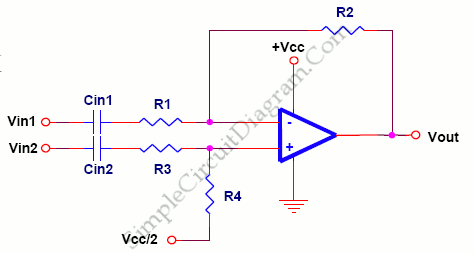# Difference Amplifier Eliminates Vocal Track

Not only a summing circuits, but there are also subtracting circuits. Here’s the circuit diagram:Subtracting circuit is a common application which is used to eliminate the vocal track (recorded at equal levels in both channels) from stereo recordings.
R1 = R3 and R2 = R4,
Vout = (R2/R1)(Vin2 – Vin1)
R1||R2 = R3||R4
for minimum error due to input bias current.  [Source: Texas Instruments Application Note]